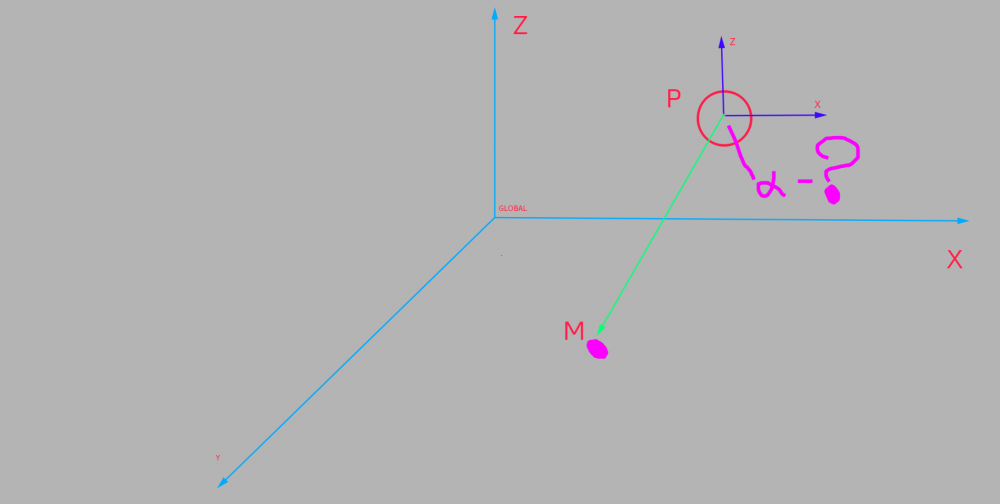# Rotation mesh

## Recommended Posts

Friends! It's a simple mathematical problem. And it can be solved. That is the question. How is it possible to implement built-in methods of Babylon

So. There is an object. In the figure it is denoted P(xp, yp).
There is a point where the object should move. M(xm, ym)

The question of how to organize the rotation of the object so that its positive X-axis was directed in the direction M when the object moves.

I mathematically to calculate the angle?

mesh.rotate(BABYLON.Axis.Y, THIS_ANGLE, BABYLON.Space.WORLD)

Or is there a method to which you can put the coordinates of the point and he will apply and I will turn my object in the desired direction?

WARNING: Rotate only around the axis Y (2D rotate)##### Share on other sites

Hi Andrey Zimin.

While depending on how you draw your mesh, you may use the method LookAt.
Here is a PG (modify line 50 to see behaviour)

##### Share on other sites

https://en.wikipedia.org/wiki/Dot_product (I think it's the cosine of that).  The formula in the Math class:
https://github.com/BabylonJS/Babylon.js/blob/master/src/Math/babylon.math.ts#L2121

I think it returns the cosine of the angle between the 2.  Only rotating on the Y, then make the second vector have the same Y?  If you make a PG I think we can figure it out.

Here's my favourite lookAt PG (I think it's from Adam):
https://www.babylonjs-playground.com/#UMQ4UR#2

## Join the conversation

You can post now and register later. If you have an account, sign in now to post with your account.
Note: Your post will require moderator approval before it will be visible.×   Pasted as rich text.   Paste as plain text instead

Only 75 emoji are allowed.